Enter Category Name
Enter Category Name
Enter Category Name
Enter Category Name
Enter Category Name
100

Which real object has 8 sides and 8 corners?

a) an ice cream cone

b) a stop sign

c) a piece of paper

b) a stop sign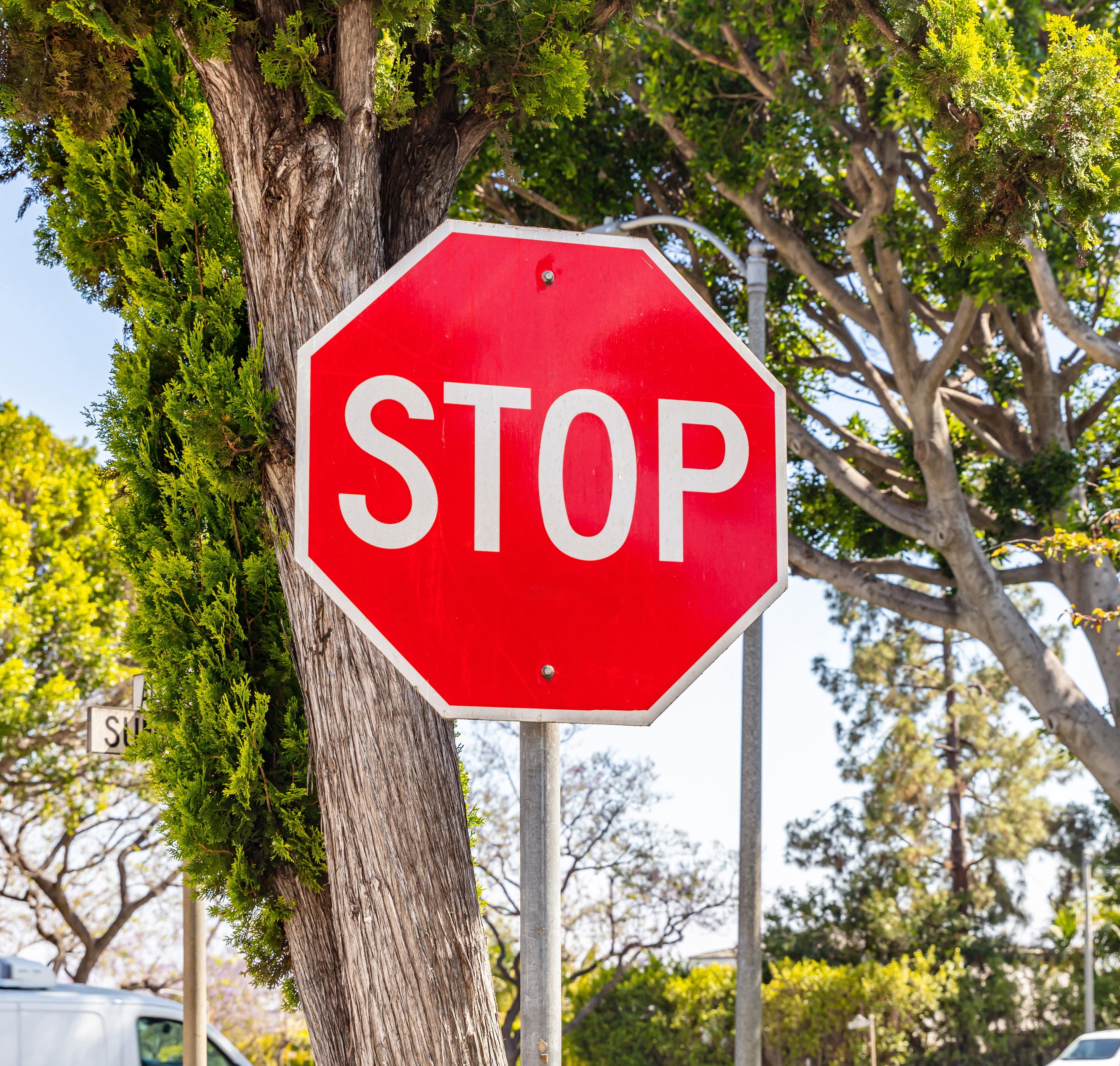100

Choose the correct symbol: <, =, >

43 ______ 12

>

100

Which is the shortest?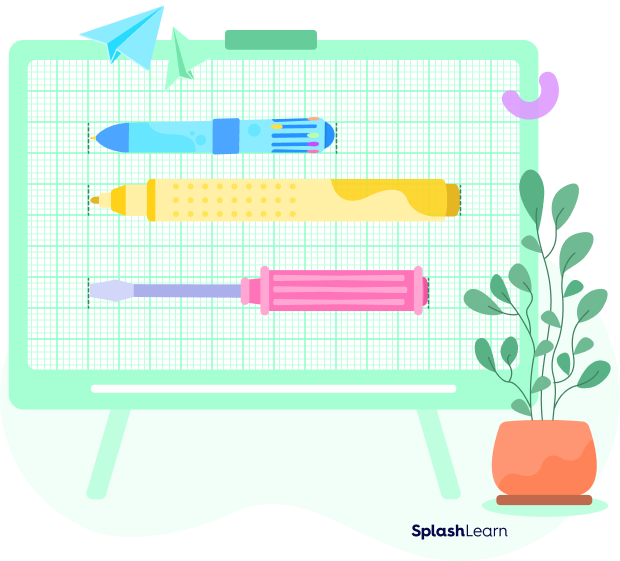The blue one

100

Which number is missing?

12, 13, _____, 15, 16

14

100

Choose the correct symbol: <, =, or >

33 ______ 33

=

200

34 + 4 = _______

38

200

What comes next in the time pattern?

3:00, 4:00, 5:00, ______

6:00

200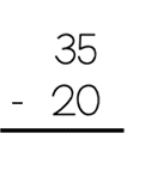15

200

Use the number line to solve.

3 + 5 = _____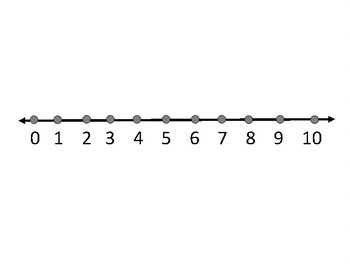8

200

What comes next in the time pattern?

1:30, 2:00, 2:30, _____

3:00

300

How do you put the following numbers in order from least to greatest: 6, 33, 31, 29, 786, 29, 31, 33, 78

300

How many groups of tens and tens are there?2 tens, 6 ones

300

How much money is 8 dimes plus 1 dime?

9 dimes = 90 cents

300

Put the following numbers in order from least to greatest: 36, 13, 63, 62, 9113, 36, 62, 63, 91

300

How much money is 2 dimes plus 2 dimes?

40 cents

400

If you put these two shapes together, what do you get?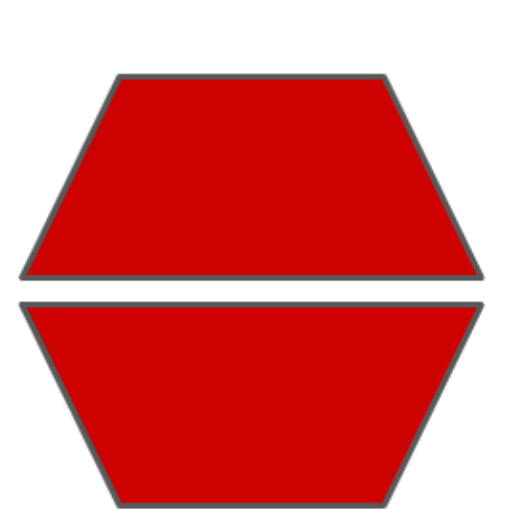Hexagon

400

Four friends share a pizza. How would you cut it so that the parts are equal?

a) cut it in half

b) cut it in thirds

c) cut it in fourths

d) don't cut it at all

c) cut it in fourths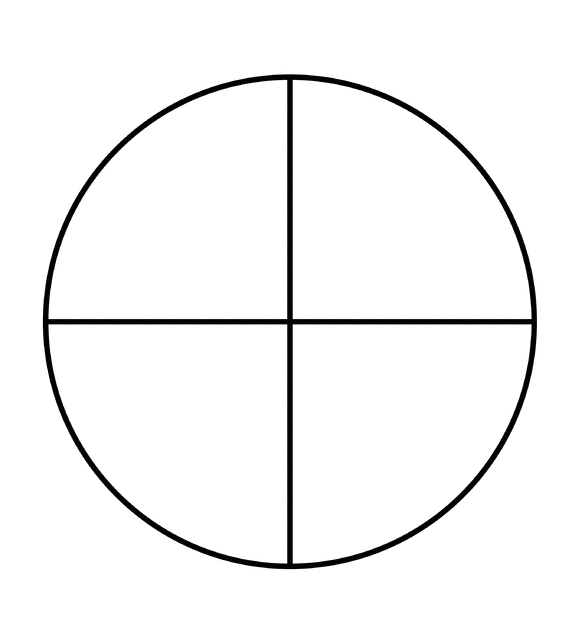400

What is the model's expanded form?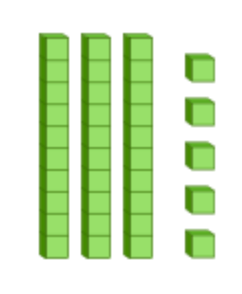30 + 5

400

What is the expanded form of the model?10 + 8

400

How many tens and ones?3 tens, 2 ones

500

How can you use subtraction to find the missing addend?

10 + ____ = 15

15 - 10 = 5

500

Layla collects 4 Pokemon cards. Travis collects 5 Pokemon cards. Write a number sentence to show how many Pokemon cards they collected in all.

4 + 5 = 9

500

How can you use subtraction to find the missing addend.

____ + 7 = 9

9 - 7 = 2

500

Luca has 30 baseball cards. Tim gives him 60 baseball cards. Write a number sentence to determine how many baseball cards Luca has now.

30 + 60 = 90

500

How can you use subtraction to find the missing addend?

4 + ____ = 8

8 - 4 = 4

Click to zoom